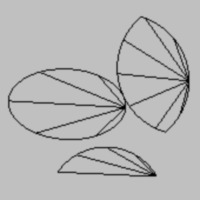# Multipoint fixture.

#### Luden

You must be logged in to obtain assets

Contact Publisher

### Description

This extension let you create fixture with more than eight points. Almost. Really you get list of fixtures, but that list so easy to use as common fixture.

How to use? First, you have to create a ds_list which contains points of your figure. Like next:

``````points_list = ds_list_create();
...
``````

Don't worry about clockwise or convex. Just create more than two points.

Second, create a list of fixture.

``````my_fixture = get_fixture(points_list);
``````

That function return list of fixture, or simple fixture(if number points of figure eight or less).

Third, add physics variable and bind. I created functions which duplicate common fixture_set_... for list of fixtures.

``````list_fixture_set_density(my_fixture, 0.8);
list_fixture_set_restitution(my_fixture, 0.1);
list_fixture_set_linear_damping(my_fixture, 0.5);
list_fixture_set_angular_damping(my_fixture, 0.5);
list_fixture_set_friction(my_fixture, 0.5);
list_fixture_set_collision_group(my_fixture, 1);
list_fixture_bind(my_fixture, id);
list_fixture_delete(my_fixture);
``````

That's all. Almost.

Also I created functions for easy creating sectors, ellipses and lenses.

``````segment_create(x, y, r, angle1, angle2, step);
``````

x, y - coordinates of centre circle r - radius angle1, angle2 - sector lies between this angles step - here and everywhere it is precision. The less step, the more precision.

``````lens_create(x, y, width, height, angle, step);
ellipse_create(x, y, width, height, angle, step);
``````

x, y - coordinates of centre figure width, height - size of figure angle - angle of rotation

Well, that's all. Definitely.